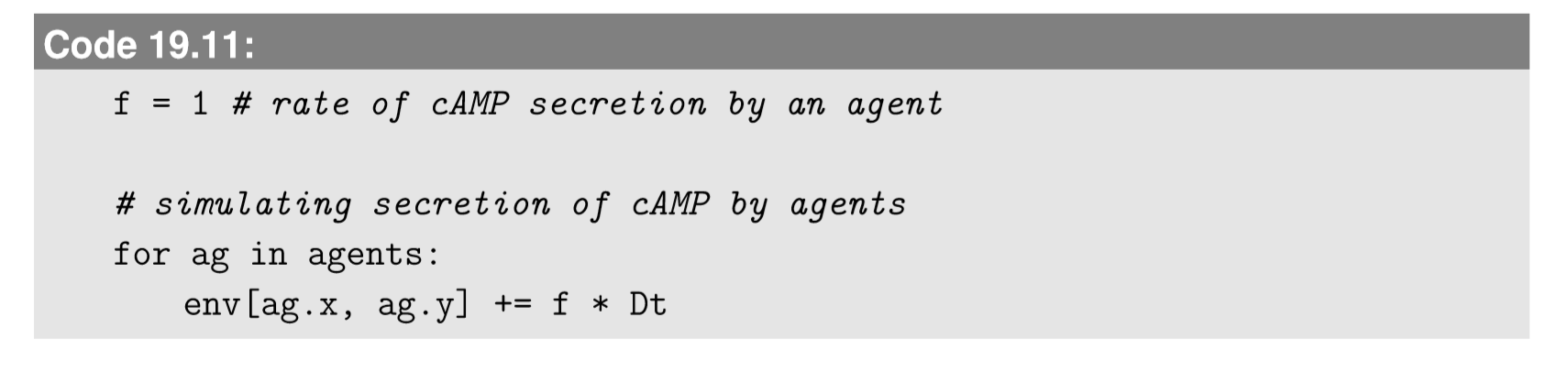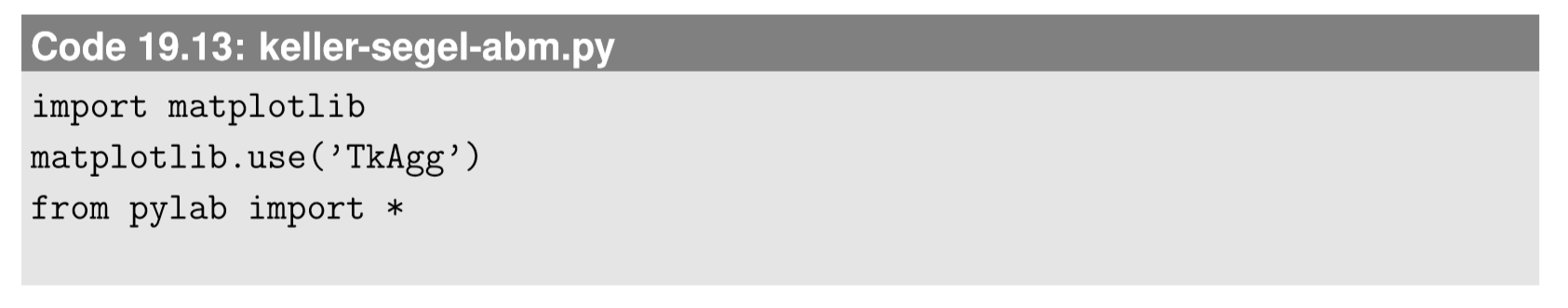Skip to main content
$$\newcommand{\id}{\mathrm{id}}$$ $$\newcommand{\Span}{\mathrm{span}}$$ $$\newcommand{\kernel}{\mathrm{null}\,}$$ $$\newcommand{\range}{\mathrm{range}\,}$$ $$\newcommand{\RealPart}{\mathrm{Re}}$$ $$\newcommand{\ImaginaryPart}{\mathrm{Im}}$$ $$\newcommand{\Argument}{\mathrm{Arg}}$$ $$\newcommand{\norm}{\| #1 \|}$$ $$\newcommand{\inner}{\langle #1, #2 \rangle}$$ $$\newcommand{\Span}{\mathrm{span}}$$

# 9.3: Agent-Environment Interaction

$$\newcommand{\vecs}{\overset { \rightharpoonup} {\mathbf{#1}} }$$

$$\newcommand{\vecd}{\overset{-\!-\!\rightharpoonup}{\vphantom{a}\smash {#1}}}$$

One important component you should consider adding to your ABM is the interaction between agents and their environment. The environmental state is still part of the system’s overall state, but it is deﬁned over space, and not associated with speciﬁc agents. The environmental state dynamically changes either spontaneously or by agents’ actions (or both). The examples discussed so far (Schelling’s segregation model, DLA, Boids) did not include such an environment, but many ABMs explicitly represent environments that agents act on and interact with. The importance of agent-environment interaction is well illustrated by the fact that NetLogo , a popular ABM platform, uses “turtles” and “patches” by default, to represent agents and the environment, respectively. We can do the same in Python.

A good example of such agent-environment interaction is in the Keller-Segel slime mold aggregation model we discussed in Section 13.4, where slime mold cells behave as agents and interact with an environment made of cAMP molecules. The concentration of cAMP is deﬁned everywhere in the space, and it changes by its own inherent dynamics (natural decay) and by the actions of agents (secretion of cAMP molecules by agents). This model can be implemented as an ABM, designed step by step as follows:

1. Design the data structure to store the attributes of the agents. If the slime mold cells are represented by individual agents, their concentration in the original Keller-Segel model is represented by the density of agents, so they will no longer have any attributes other than spatial position in the 2-D space. Therefore x and y are the only attributes of agents in this model.

2. Design the data structure to store the states of the environment. The environment in this model is the spatial function that represents the concentration of cAMP molecules at each location. We can represent this environment by discretizing space and assigning a value to each discrete spatial cell, just like we did for numerical simulations of PDEs. We can use the array data structure for this purpose.

Here is a sample initialize part that sets up the data structures for both agents and the environment. Note that we prepare two arrays, env and nextenv, for simulating the dynamics of the environment.3. Describe the rules for how the environment behaves on its own. The inherent dynamics of the environment in this model are the diffusion and spontaneous decay of the cAMP concentration. These can be modeled by using the discrete version of the Laplacian operator, as well as an exponential decay factor, applied to the environmental states everywhere in the space in every iteration. This is no different from what we did for the numerical simulations of PDEs. We can implement them in the code as follows:Here we adopt periodic boundary conditions for simplicity.

4. Describe the rules for how agents interact with the environment. In this model, agents interact with the environment in two different ways. One way is the secretion of cAMP by the agents, which can be implemented by letting each agent increase the cAMP concentration in a discrete cell where it is located. To do this, we can add the following to the update function:The other way is the chemotaxis, which can be implemented in several different ways. For example, we can have each agent look at a cell randomly chosen from its neighborhood, and move there with a probability determined by the difference in cAMP concentration ($$∆c$$) between the neighbor cell and the cell where the agent is currently located. A sigmoid function
$P ( \Delta c ) = \frac { e ^ { \Delta c / c _ { 0 } } } { 1 + e ^ { \Delta c / c _ { 0 } } } \label{(19.1)}$

would be suitable for this purpose, where $$c_{o}$$ is a parameter that determines how sensitive this probability is to $$∆c$$. $$P(∆c)$$ approaches 1 with $$∆c → ∞$$, or 0 with $$∆c → −∞$$. This part can be implemented in the update function as follows:Here diff corresponds to $$∆c$$, and we used $$c_0 = 0.1$$.

5. Describe the rules for how agents behave on their own. All the actions taken by the agents in this model are interactions with the environment, so we can skip this design task.

6. Describe the rules for how agents interact with each other. In this model, agents don’t interact with each other directly; all interactions among them are indirect, mediated by environmental variables (cAMP concentration in this case), so there is no need to implement anything for the agents’ direct interaction with each other. Indirect agent-agent interaction through informational signals written in the environment is called stigmergy, which is an essential coordination mechanism used by many social organisms .

By putting everything together, and adding the observe function for visualization, the entire simulator code looks like this:As you see, the code is getting longer and more complicated than before, which is a typical consequence when you implement an ABM.

Figure 19.3.1 shows a typical simulation result. The tiny blue dots represent individual cells (agents), while the grayscale shades on the background show the cAMP concentration (environment). As time goes by, the slightly more populated areas produce more cAMP, attracting more cells. Eventually, several distinct peaks of agent populations are spontaneously formed, reproducing self-organizing patterns that are similar to what the PDE-based model produced.

What is unique about this ABM version of the Keller-Segel model, compared to its original PDE-based version, is that the simulation result looks more “natural”—the formation of the population peaks are not simultaneous, but they gradually appear one after another, and the spatial arrangements of those peaks are not regular. In contrast, in the PDE-based version, the spots are formed simultaneously at regular intervals (seeFigure $$\PageIndex{1}$$: Visual output of Code 19.13. Time ﬂows from left to right, then from top to bottom.

Fig. 13.4.3). Such “natural-lookingness” of the results obtained with ABMs comes from the fact that ABMs are based on the behaviors of discrete individual agents, which often involve a lot of realistic uncertainty.

Exercise $$\PageIndex{1}$$

Conduct simulations of the ABM version of the Keller-Segel model with k, Dc, and f varied systematically. Are the effects of those parameters on pattern formation similar to those in the PDE version of the same model (see Eq. (14.3.40))?

Exercise $$\PageIndex{2}$$

Implement an additional mechanism into the ABM above to prevent agents from aggregating too densely at a single location, so that the population density doesn’t exceed a predetermined upper limit anywhere in the space. Conduct simulations to see what kind of effect this additional model assumption has on pattern formation.

Exercise $$\PageIndex{3}$$

Garbage collection by ants This is another interesting ABM with an agent-environment interaction, which was ﬁrst presented by Mitchel Resnick in his famous book “Turtles, Termites and Trafﬁc Jams” . Assume there are many tiny pieces of garbage scattered in a 2-D space, where many ants are wandering randomly. When an ant comes to a place where there is some garbage, it behaves according to the following very simple rules:

1. If the ant is holding a piece of garbage, it drops the piece.

2. If the ant isn’t holding any garbage, it picks up a piece of garbage.

What would result from these rules? Are the garbage pieces going to be scattered more and more due to these brainless insects? Implement an ABM of this model and conduct simulations to ﬁnd out what kind of collective behavior emerges.

If you implement the model right, you will see that these very simple behavioral rules let the ants spontaneously collect and pile up garbage and clear up the space in the long run. This model tells us how such emergent behavior of the collective is sometimes counter to our natural intuition.